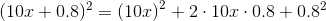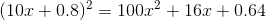# PSAT Math : How to find the square of a sum

## Example Questions

### Example Question #1 : Square Of Sum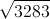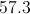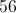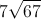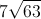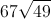Explanation:

We can break the square root down into 2 roots of 67 and 49. 49 is a perfect square and reduces to 7.

### Example Question #1 : How To Find The Square Of A Sum

Expand: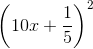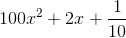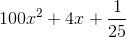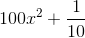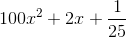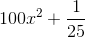Explanation:

Use the perfect square trinomial pattern, setting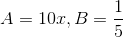: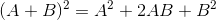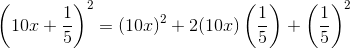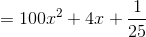### Example Question #2 : How To Find The Square Of A Sum

If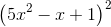is expanded, what is the coefficient of?There is noterm in the expansion of.Explanation: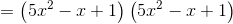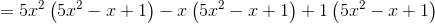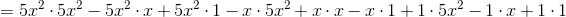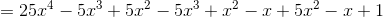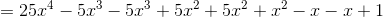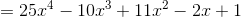The coefficient ofis therefore 11.

### Example Question #3 : How To Find The Square Of A Sum

Ifis expanded, what is the coefficient of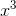?

There is noterm in the expansion of.Explanation:The coefficient ofis therefore 10.

### Example Question #4 : How To Find The Square Of A Sum

Expand: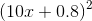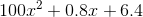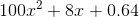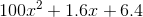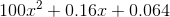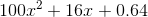Explanation:

Use the perfect square trinomial pattern, setting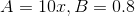: